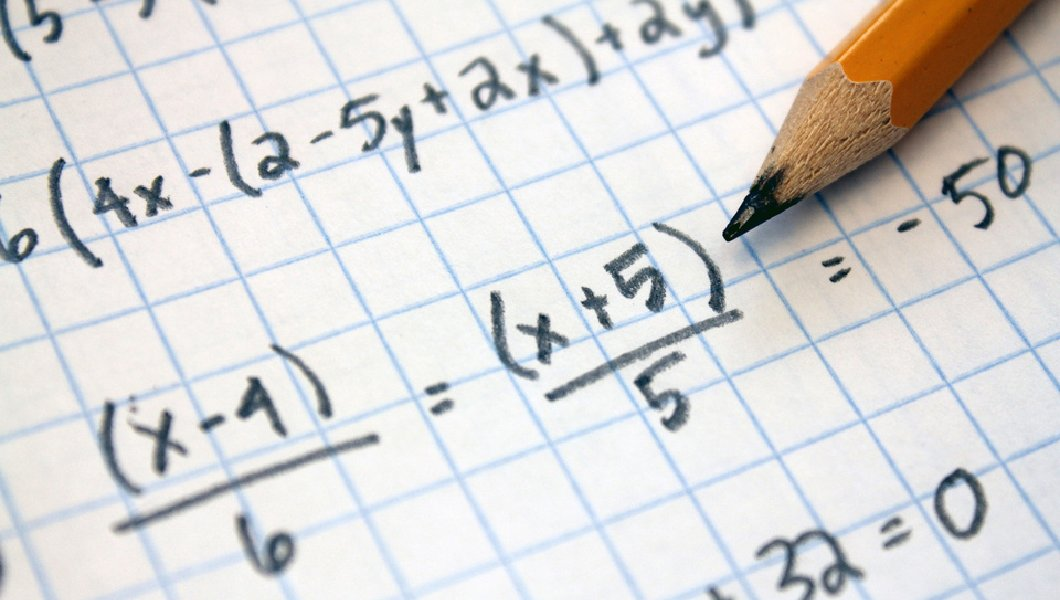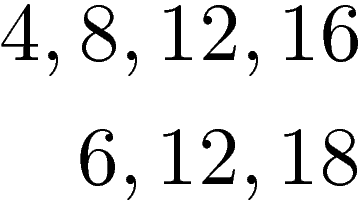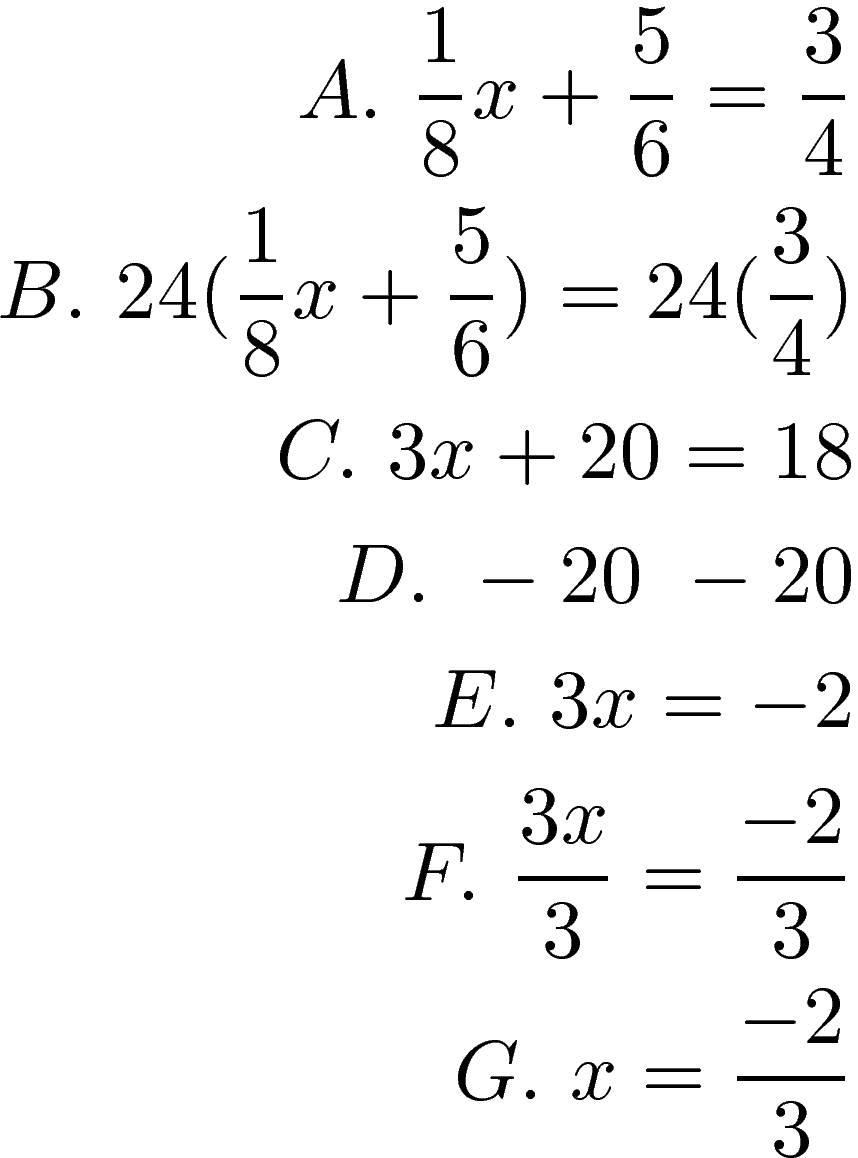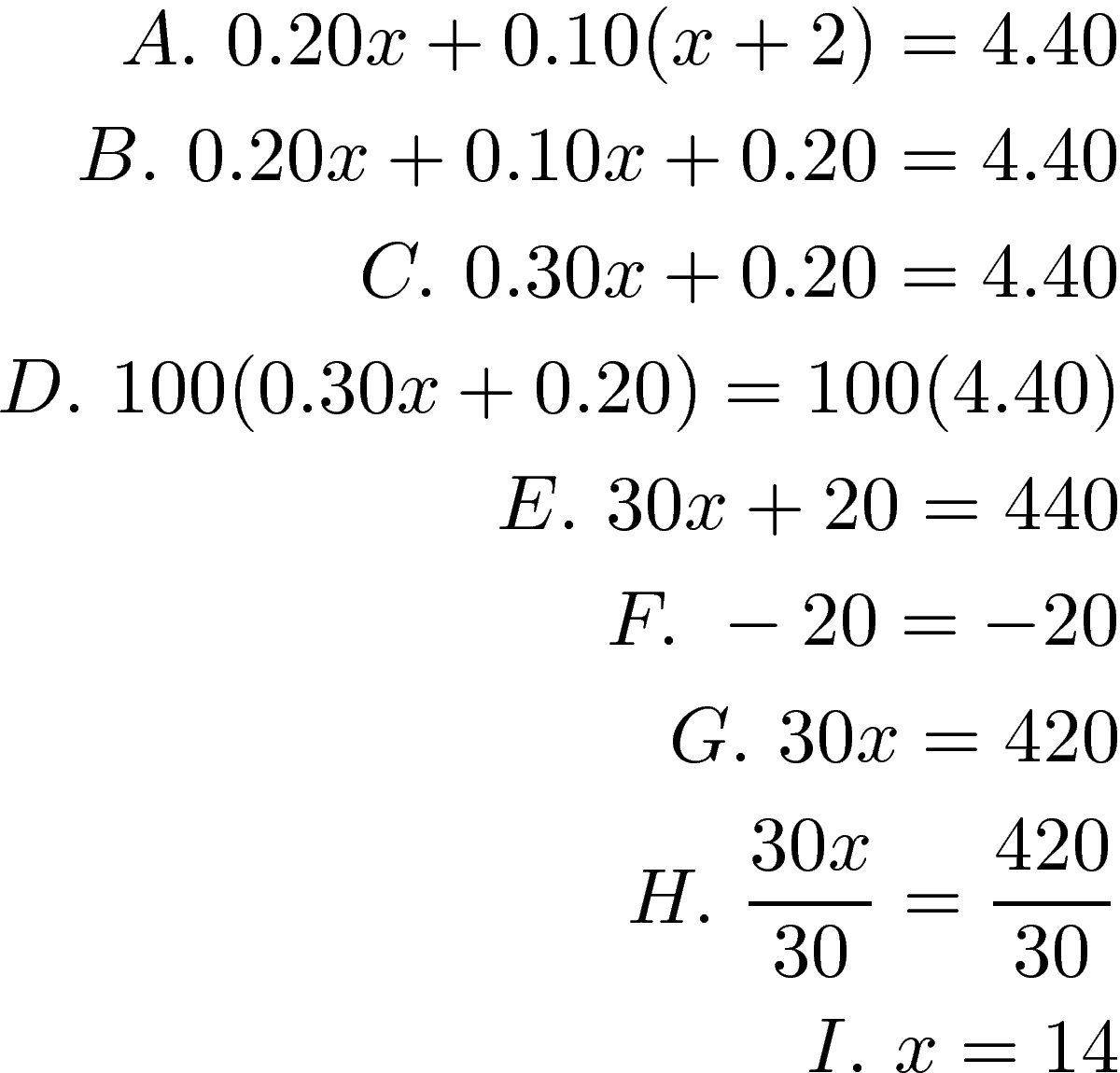# Solving Equations with Fractions or Decimals

This post will provide an explanation of how to solve equations that include fractions or decimals. The processes are similar in that both involve determining the least common denominator.Solving Equations with Fractions

The key step to solving equations with fractions is to make sure that the denominators of all the fractions are the same. This can be done by finding the least common denominator. The least common denominator (LCD) is the smallest multiple of the denominators. For example, if we look at the multiples of 4 and 6 we see the following.You can see clearly that the number 12 is the first multiple that 4 and 6 have in common. You can find the LCD by making factor trees but that is beyond the scope of this post. The primary reason we would need the LCD is when we are adding fractions in an equation. If we are multiplying we could simply multiply straight across.

Below is an equation that has fractions. We will find the LCDHere is an explanation of each step

• A. This is the original problem. We first need to find the LCD
• B. We then multiply each fraction by the LCD
• C. This is the equation we solve for
• D. We get the variable alone by subtracting 20 from each side
• E. We have our new simplified equation
• F. We further isolate the variable by dividing by 3 on both sides.
• G. This is our answer

Solving Equations with Decimals

The process for solving equations with decimals is almost the same as for fractions. The LCD of all decimals is 100. Therefore, one common way to deal with decimals is to multiply all decimals by 100 and the continue to solve the equation.

The primary benefit of multiply by 100 is to remove the decimals because sometimes we make mistakes with where to place decimals. Below is an example of an equation with decimals.￼Here is what we did

• A. Initial equation
• B. we distribute the 0.10
• C. Revised equation
• D. We multiply everything by 100.
• E. Revised equation
• F. Subtract 20 from both sides to isolate the variable
• G. Revised equation
• H. Divide both sides by 30 to isolate the variable